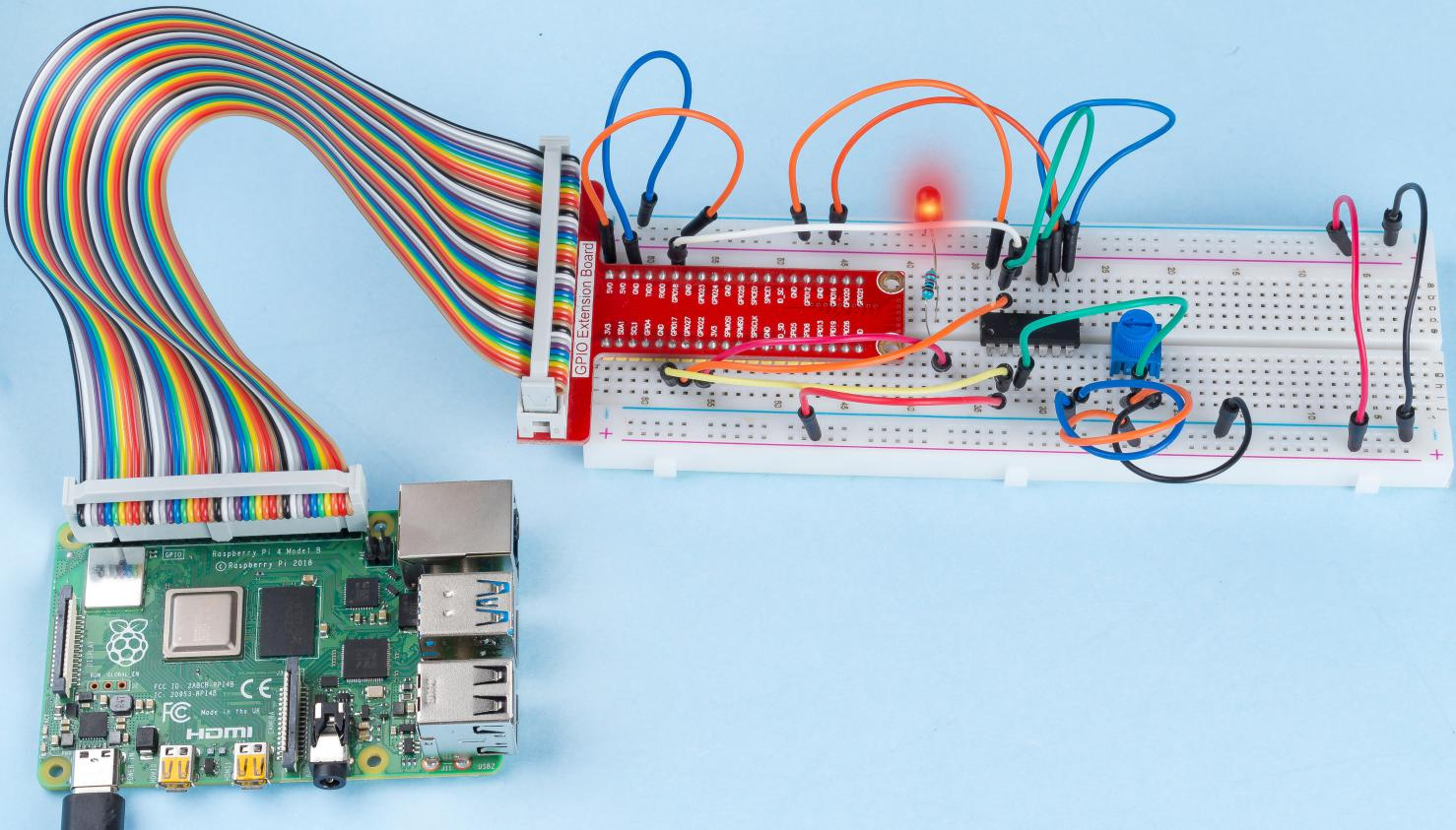# 2.1.4 Potentionmeter¶

## Introduction¶

The ADC function can be used to convert analog signals to digital signals, and in this experiment, ADC0834 is used to get the function involving ADC. Here, we implement this process by using potentiometer. Potentiometer changes the physical quantity – voltage, which is converted by the ADC function.

## Components¶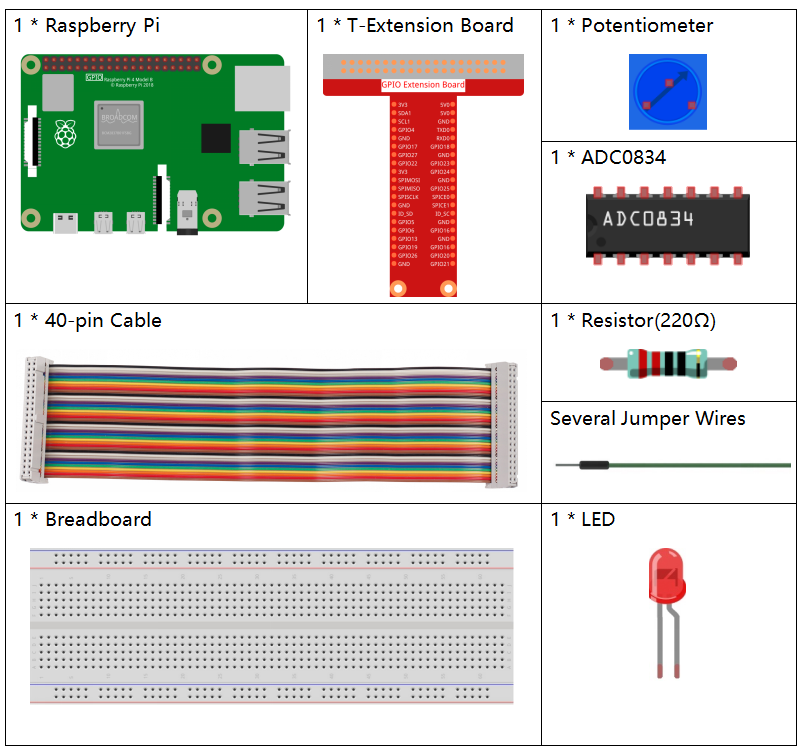## Schematic Diagram¶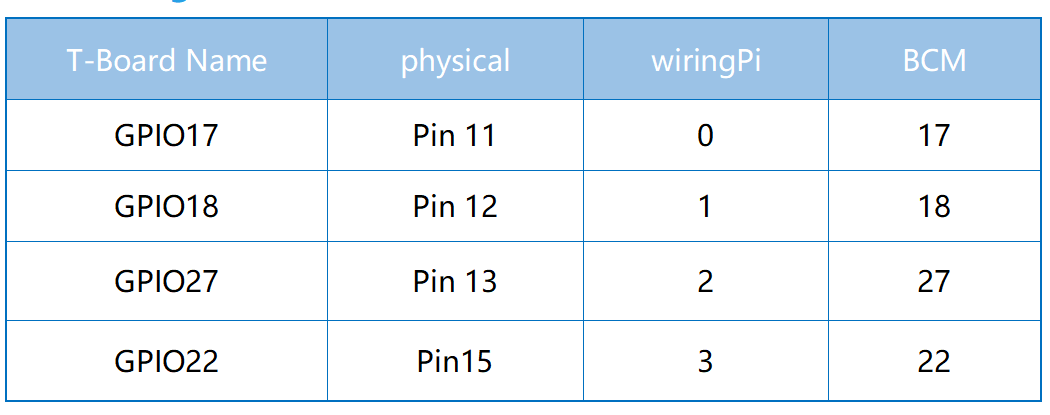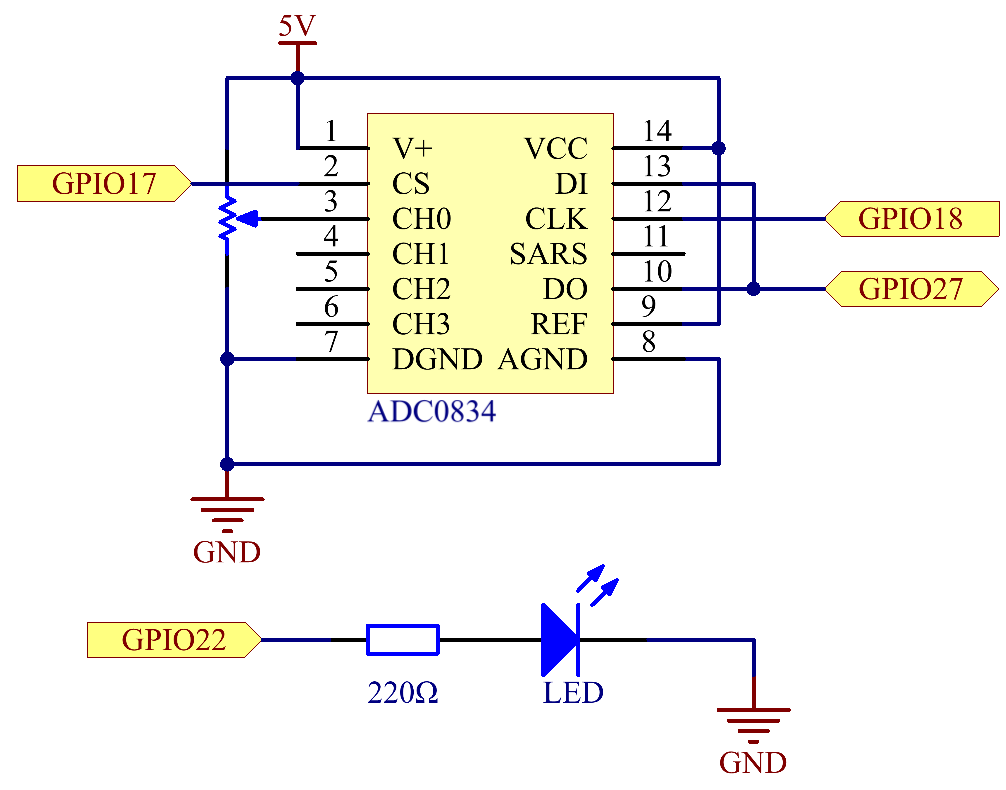## Experimental Procedures¶

Step 1: Build the circuit.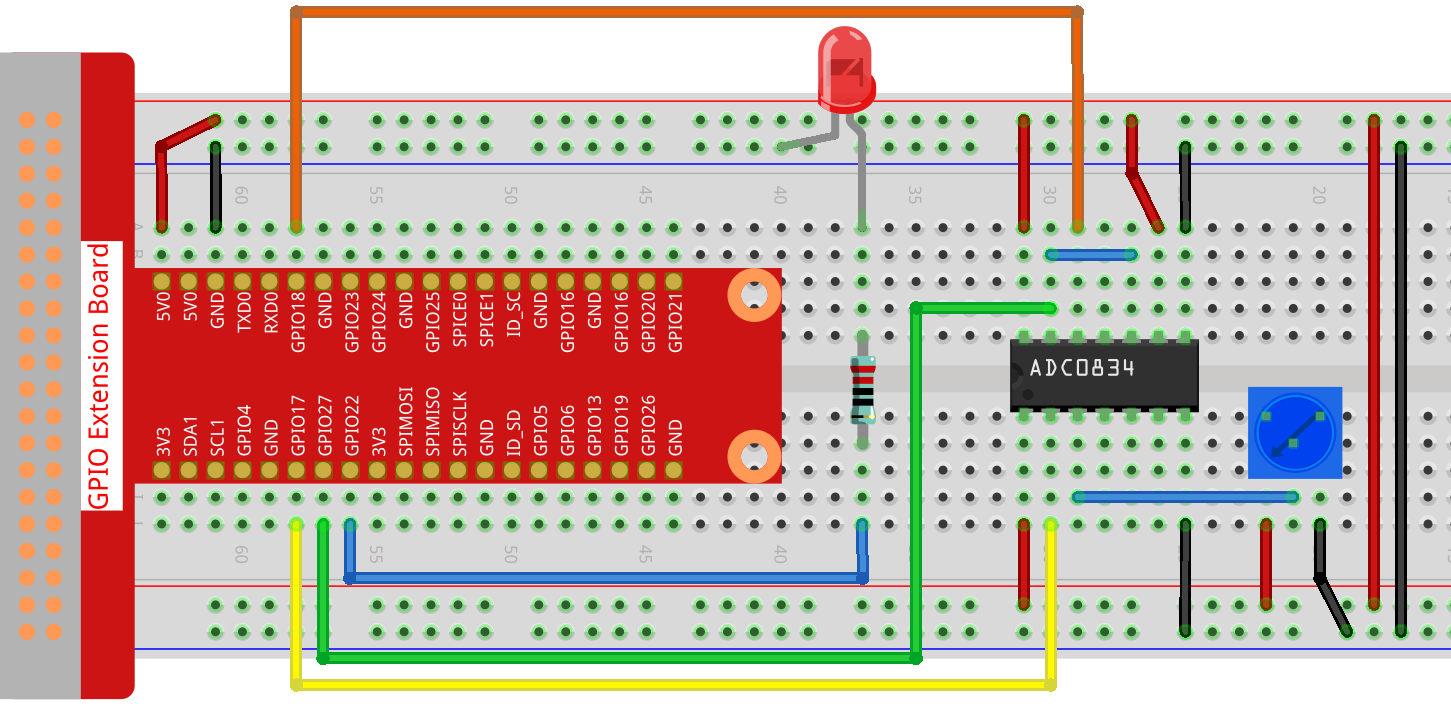Note

Please place the chip by referring to the corresponding position depicted in the picture. Note that the grooves on the chip should be on the left when it is placed.

Step 2: Go to the folder of the code.

```cd /home/pi/davinci-kit-for-raspberry-pi/nodejs/
```

Step 3: Run the code.

```sudo node potentionmeter.js
```

After the code runs, rotate the knob on the potentiometer, the intensity of LED will change accordingly.

Code

```const Gpio = require('pigpio').Gpio;

const led = new Gpio(22, {mode: Gpio.OUTPUT});

setInterval(() => {
console.log(`Current analogVal: \${value}\n`);
led.pwmWrite(value);
}, (error)=>{
console.log("Error: " + error);
});
}, 100);
```

Code Explanation

```const Gpio = require('pigpio').Gpio;
```

Import the `pigpio` module.

```const ADC0834 = require('./adc0834.js').ADC0834;
```

We import an `ADC0834` constructor to use the adc0834 module.

```const adc = new ADC0834(17, 18, 27);
```

Instantiate an ADC0834 object, the three parameters are its three pins.

This is a promise object, you may need to understand the concept from the following link.

```setInterval(() => {
console.log(`Current analogVal: \${value}\n`);
led.pwmWrite(value);
}, (error)=>{
console.log("Error: " + error);
});
}, 100);
```

The value of ADC0834 channel 0 (channel 0 is connected to the potentiometer) is read every 100ms, and the value will be stored in `value`.

Print `value` and use it to control the brightness of the LED, now you can see that the brightness of the LED changes with the value of the potentiometer.

## Phenomenon Picture¶